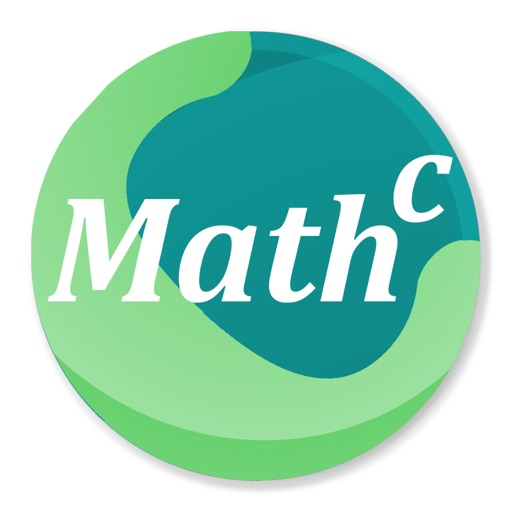## Math-c is a light and simple math script application and interactive environment for mathematical calculation, programming, plotting and image processing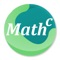# Math-c

by AutoQ3D Team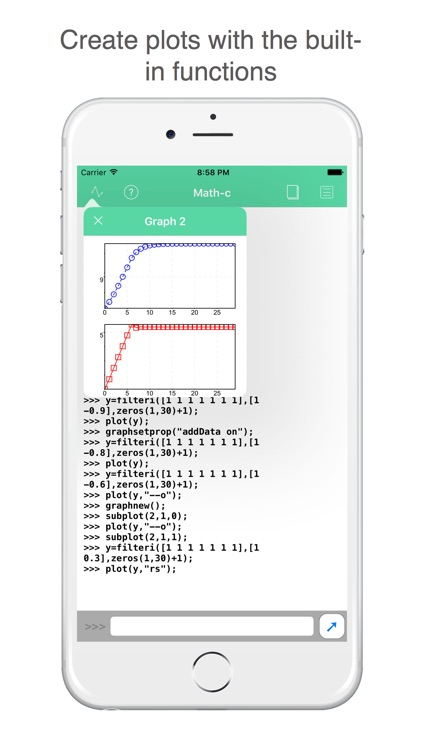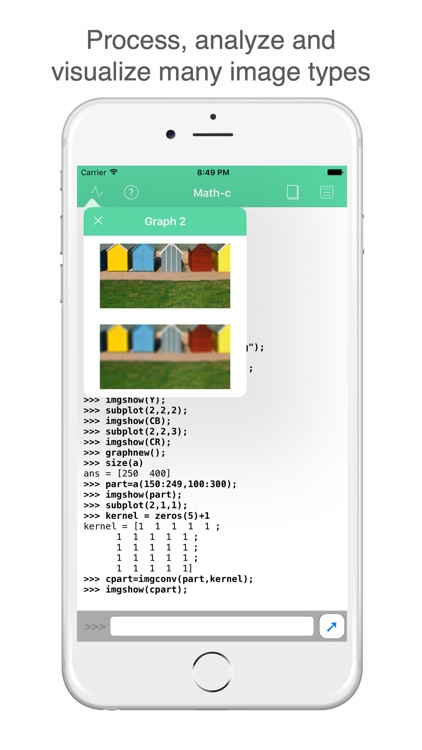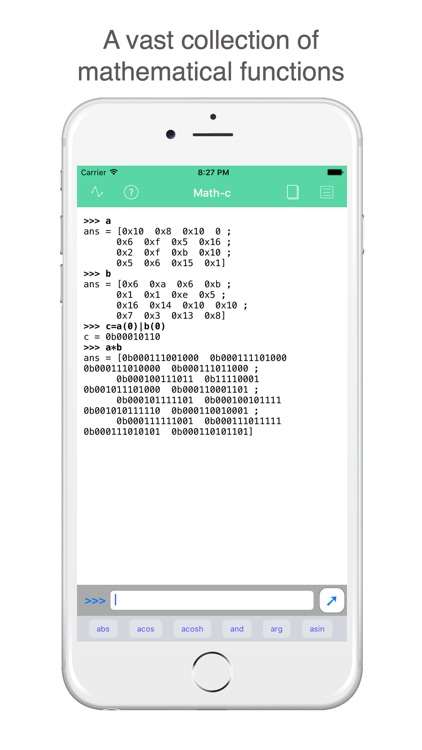Math-c is a light and simple math script application and interactive environment for mathematical calculation, programming, plotting and image processing.### App Details

Version
1.2.4
Rating
NA
Size
4Mb
Genre
Productivity Education
Last updated
July 20, 2021
Release date
October 4, 2016

### App Screenshots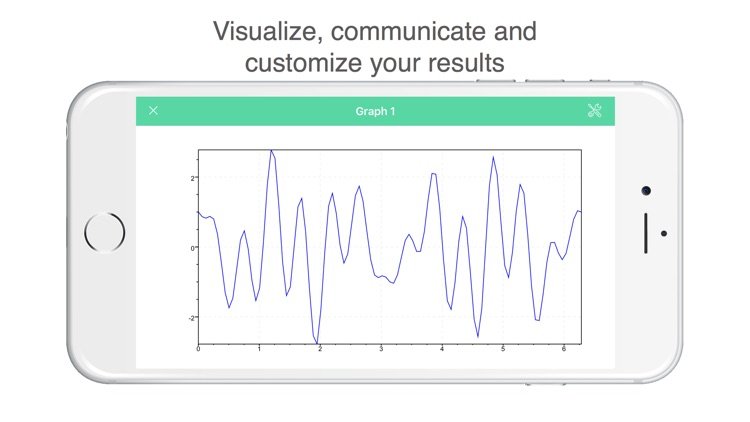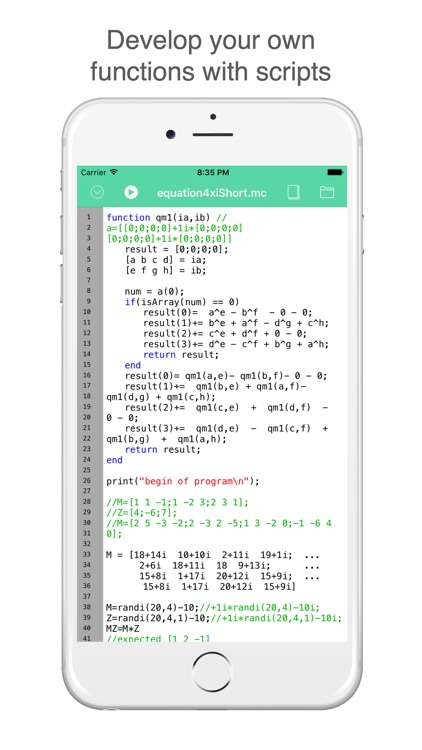### App Store Description

Math-c is a light and simple math script application and interactive environment for mathematical calculation, programming, plotting and image processing.

Features
• It allows you to create nested structures and script programs
• It allows you to use functions for linear algebra.
• It provides built-in graphics to analyze data and options to customize these plots.

Uses of Math-c mathematical calculations
Math-c can be used for many mathematical calculations like the following:
• Arrays
• Matrices
• 2D and graphics
• Linear Algebra
• Quaternions
• Complex numbers
• Work in hexadecimal and binary format

Areas where Math-c can be of benefit
Math-c can be used as a simple tool in math, programming, chemistry, physics, engineering and in applications as the following:
• Signal Processing and Communications
• Image Processing
• Fourier analysis
• Mathematical Calculations

Learning and documentation
・Documentation section in the website.
・Email support

We appreciate your positive reviews into the App Store and your feedback to [email protected] so you can help us give you a better service.

Disclaimer:
AppAdvice does not own this application and only provides images and links contained in the iTunes Search API, to help our users find the best apps to download. If you are the developer of this app and would like your information removed, please send a request to [email protected] and your information will be removed.